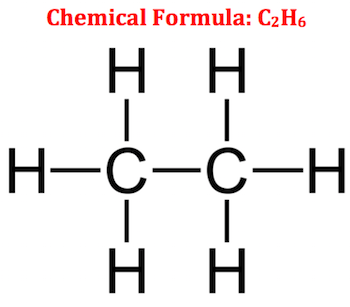# Question 74d95

Feb 19, 2017

Ethane has 14 valence electrons.

#### Explanation:

The formula of ethane is ${\text{C"_2"H}}_{6}$.

Each $\text{C}$ atom has 4 valence electrons and each $\text{H}$ atom has 1 valence electron.

∴ A molecule of ${\text{C"_2"H}}_{6}$ has 2×4 + 6×1= 8 + 6 = 14# valence electrons.

You can count them in the structure of ethane.Each line represents a bond (a pair of electrons).

The 7 bonds contain 14 valence electrons.